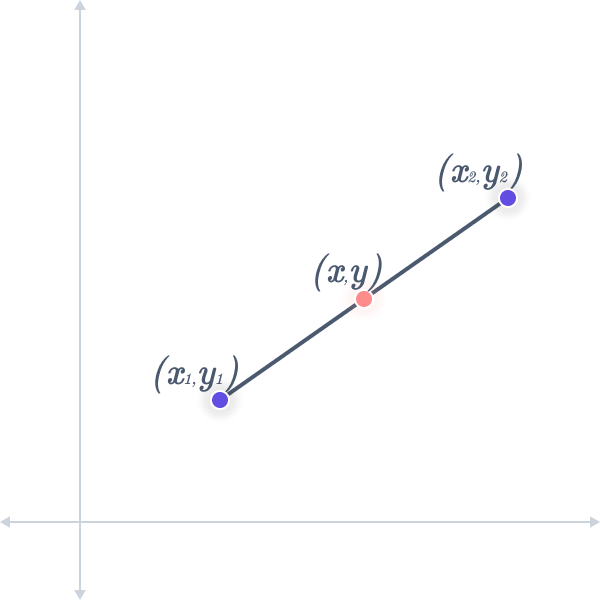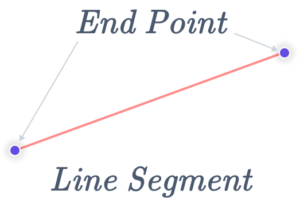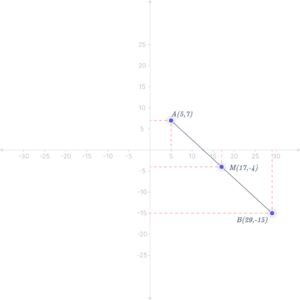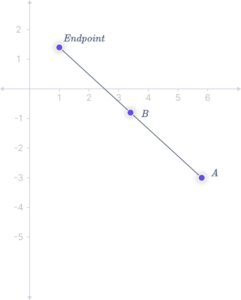# Endpoint Calculator

An online endpoint calculator can accurately determine the endpoint of a line segment based on the provided starting and midpoints. With this convenient endpoint calculator, all you need to input are the start and midpoints, and it will swiftly compute and reveal the endpoint with precision and ease. If you're pressed for time, feel free to skip the detailed definition of an endpoint and scroll down to find the endpoint calculator below.Starting point coordinates

Midpoint coordinates

## What is an Endpoint?

An endpoint in geometry is a point that identifies a section of a straight line. For example, a segment is uniquely defined by two points, A and B, with a single point that bisects it, such as M (middle point).

A line segment’s endpoint is any point at either end. A line in a line segment does not extend past its endpoints. It is therefore described as a point where a line finishes.## Endpoint Formula

To derive the endpoint from the midpoint and other endpoints, we can use this formula

$$x_2 = 2x – x_1$$

$$y_2 = 2y – y_1$$

Where,

• $$(x_2, y_2)$$ are the coordinates of the endpoint
• $$(x_1, y_1)$$ are the starting point coordinate
• (x, y) are the coordinates of the midpoint.

## How to Find the Endpoint of a Line Segment?

We can easily determine the other endpoint when we have one endpoint and the midpoint of a line segment. We can follow a simple procedure to find the missing endpoint by leveraging the midpoint coordinates and the known endpoint. To discover the missing endpoint, follow these steps:

• Calculate the distance between the known endpoint and the midpoint.
• Measure the same distance from the midpoint in the opposite direction to find the other endpoint.

By applying this method, we can confidently pinpoint the coordinates of the missing endpoint. This approach leverages the relationship between the midpoint and the known endpoint, allowing us to deduce the location of the other endpoint accurately.

## Examples Using Endpoint Formula

Example 1: Point A is (5, 7). Point B is (x, y). The midpoint of AB is (17, –4). Next, find the endpoints of the line segment.

Solution: Point A is (5, 7). Point B is (x, y). The midpoint of AB is (17, –4). What is the value of B?

We need to use the midpoint formula which is $$\dfrac{x_1 + x_2}{2}, \dfrac{y_1 + y_2}{2}$$.

$$MP = ( \dfrac{5 + x}{2}, \dfrac{7 + y}{2} )$$

Solving each of them separately, we get:

$$\dfrac{5 + x}{2} = 17 → 5 + x = 34 → x = 29$$

$$\dfrac{7 + y}{2} = –4 → 7 + y = –8 → y = –15$$

B is (29, –15).Example 2: Find the missing endpoint of a segment with a midpoint at coordinates (2.5, -1.8) and an endpoint at coordinates (4.1, -4.2).

Solution: In the given example, the coordinates of the midpoint ($$x_m$$, $$y_m$$) are provided as (2.5, -1.8), and the coordinates of one endpoint ($$x_1$$, $$y_1$$) are given as (4.1, -4.2). We need to find the coordinates of the missing endpoint ($$x_2$$, $$y_2$$).

($$x_m$$, $$y_m$$) = (2.5, -1.8)

($$x_1$$, $$y_1$$) = (4.1, -4.2)

To find the missing endpoint, we can use the endpoint formula:

$$x_2 = 2(x_m) – x_1$$ Substituting the known values:

$$x_2 = 2(2.5) – 4.1$$

$$x_2 = 5 – 4.1$$

$$x_2 = 0.9$$

$$y_2 = 2(y_m) – y_1$$ Substituting the known values:

$$y_2 = 2(-1.8) – (-4.2)$$

$$y_2 = -3.6 + 4.2$$

$$y_2 = 0.6$$

Missing endpoint: (0.9, 0.6)## Endpoints and Midpoints

• Endpoints are the locations along a segment where a line terminates.
• Midpoints are the exact center of the line segment, located where the endpoints are.

The midpoint and the endpoint formula are connected. A midpoint is a location in the center of a line connecting two points. The midpoint formula can determine the other midpoint given one endpoint and a midpoint. Both midpoint and endpoint calculators are available on the internet for easy calculations.

## FAQs

How many endpoints are there on a line?

Since only one line may travel through a given pair of points, lines are considered two points. A line segment has two endpoints, and a line has no endpoints.

In geometry, how do you locate the endpoint?

• Draw the line segment between the starting point (A) and the midpoint (B)
• Draw a line from B away from A in an endless direction.
• Calculate the distance between places A and B, then record the distance from B traveling in the other direction. The marked point represents the goal endpoint.

How do we define the midpoint of a line segment in mathematics using endpoints?

The midpoint of a line segment is defined as the point that lies precisely equidistant between the two endpoints.

Can you determine both endpoints of a line segment if the midpoint is given?

No, to determine the endpoints, you need at least one endpoint and the midpoint coordinates.